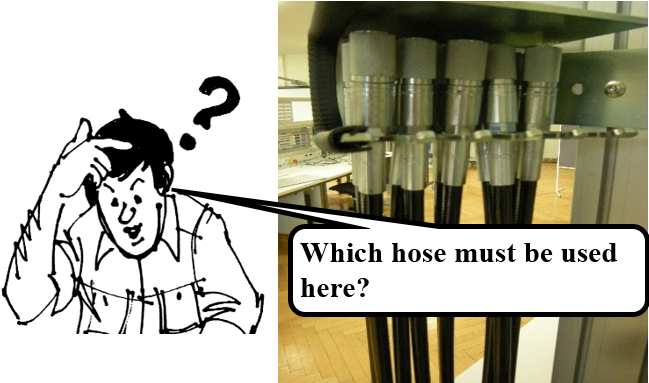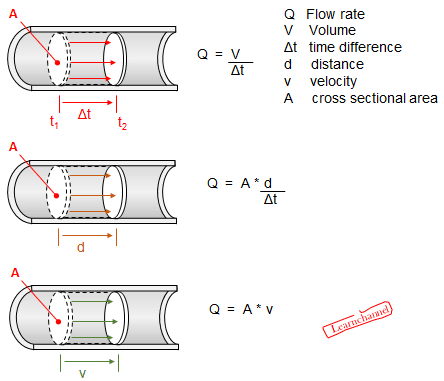# Hidrodinámica

(English)

## Laws of hydrodynamics and their application

The flow rate Q is defined to be the volume of fluid passing by some location through an area during a period of time. In symbols, this can be written as:      Q = V / t

where V is the volume and t is the elapsed time. The units you would use in hydraulics for the volume flow are liter per minute or l/min.The diameter of an oil line depends primarily on the volume flow as well as the speed of an hydraulik cylinder. In order to answer these questions, we derive the following relation:Definition of the Volume Flow Rate

Regarding a hose you see that flow rate and velocity are related. But flow rate also depends on the diameter of the hose. The precise relationship between flow rate Q and velocity v is:   Q = dV /dt = A * v

A cross sectional area,  V volume of the oil, v velocity oil

A brief overview of the flow velocities in different pipe sections:
Suction lines: 0.5 to 0.8 m / s
Return line: 2 to 4 m / s
Suction line with inlet: up to 1.4 m / s
Pressure line up to 100 bar: 2 to 4 m / s
Pressure line up to 315 bar: 3 to 12 m / s

### Equation of continuity

The following figure shows oil flowing along a pipe of decreasing radius. Because oil, like other fluids is incompressible, the same amount of fluid must flow past any point in the tube in a given time. Here the cross-sectional area of the pipe decreases, so the velocity must necessarily increase. In general you can say that the flow rate must be the same at all points along the pipe:

This is called the equation of continuity and is valid for any incompressible fluid. A nozzle is one appliance of this equation: it emerges with a large speed—that is the purpose of the nozzle.Hydraulics - Continuity Equation explained

Q1 = A1 * v1   ¦   Q2 = A2 * v2

since Q1  =  Qfollows:           A1 * v1 = A2 * v2

Throttles as hydraulic components, can reduce the oil flow by their hydraulic resistance. By using these components you can, for example, reduce the piston velocity.

2A4: The velocity of a fluid in a tube with a cross sectional area of 23 cm2 is 1.7 m/s. Which velocity you get at a cross section constriction of 10 cm2?